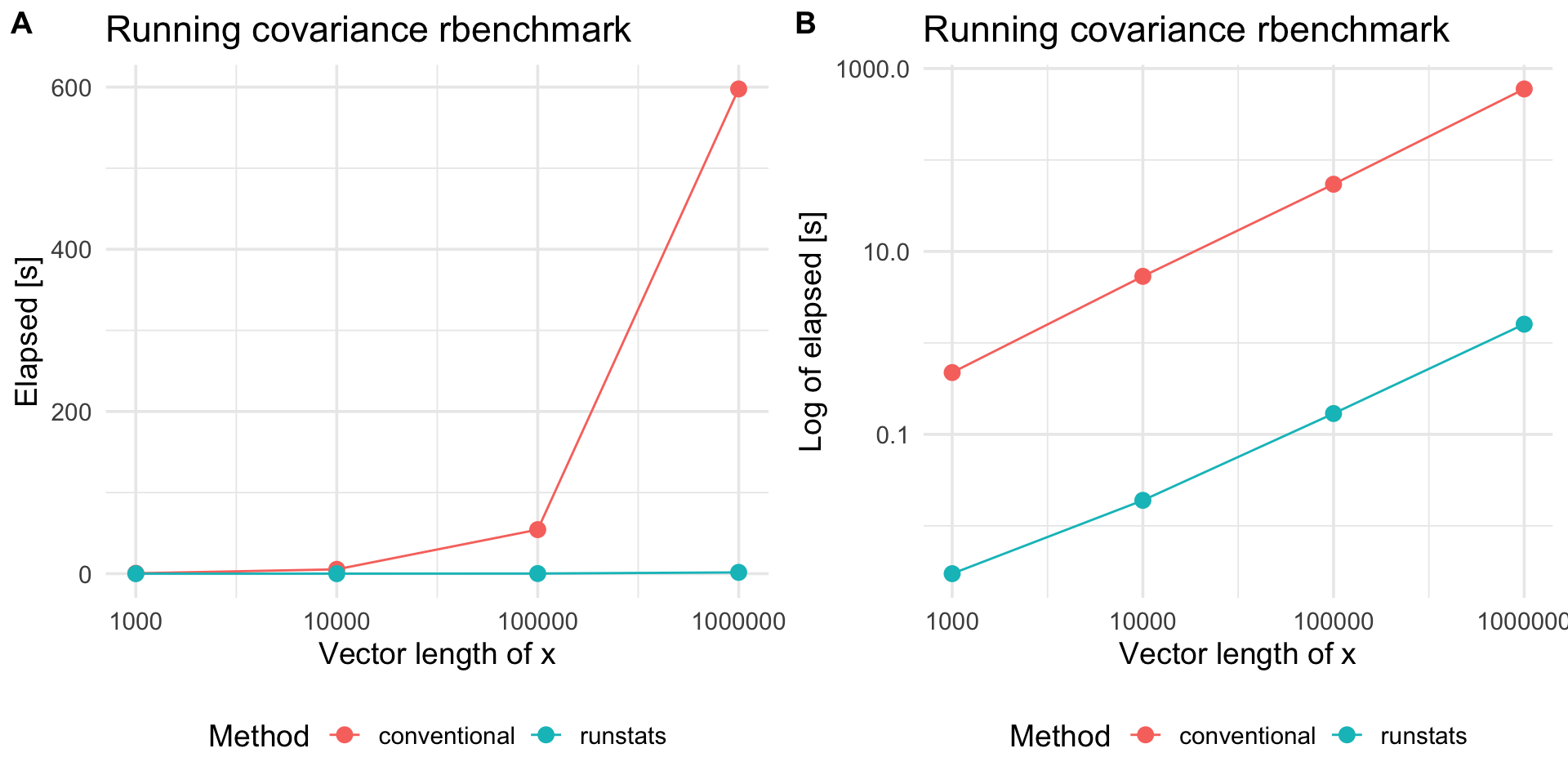# runstats

Package `runstats` provides methods for fast computation of running sample statistics for time series. The methods utilize Convolution Theorem to compute convolutions via Fast Fourier Transform (FFT). Implemented running statistics include:

1. mean,
2. standard deviation,
3. variance,
4. covariance,
5. correlation,
6. euclidean distance.

### Website

Package website is located here.

### Installation

``````# devtools::install_github("martakarass/runstats")
install.packages("runstats")``````

### Usage

``````library(runstats)

## Example: running correlation
x0 <- sin(seq(0, 2 * pi * 5, length.out = 1000))
x  <- x0 + rnorm(1000, sd = 0.1)
pattern <- x0[1:100]
out1 <- RunningCor(x, pattern)
out2 <- RunningCor(x, pattern, circular = TRUE)

## Example: running mean
x <- cumsum(rnorm(1000))
out1 <- RunningMean(x, W = 100)
out2 <- RunningMean(x, W = 100, circular = TRUE)``````

### Running statistics

To better explain the details of running statistics, package’s function `runstats.demo(func.name)` allows to visualize how the output of each running statistics method is generated. To run the demo, use `func.name` being one of the methods’ names:

1. `"RunningMean"`,
2. `"RunningSd"`,
3. `"RunningVar"`,
4. `"RunningCov"`,
5. `"RunningCor"`,
6. `"RunningL2Norm"`.
``````## Example: demo for running correlation method
runstats.demo("RunningCor")````````````## Example: demo for running mean method
runstats.demo("RunningMean")``````### Performance

We use `rbenchmark` to measure elapsed time of `RunningCov` execution, for different lengths of time-series `x` and fixed length of the shorter pattern `y`.

``````library(rbenchmark)

set.seed (20181010)
x.N.seq <- 10^(3:7)
x.list  <- lapply(x.N.seq, function(N) runif(N))
y <- runif(100)

## Benchmark execution time of RunningCov
out.df <- data.frame()
for (x.tmp in x.list){
out.df.tmp <- benchmark("runstats" = runstats::RunningCov(x.tmp, y),
replications = 10,
columns = c("test", "replications", "elapsed",
"relative", "user.self", "sys.self"))
out.df.tmp\$x_length <- length(x.tmp)
out.df.tmp\$pattern_length <- length(y)
out.df <- rbind(out.df, out.df.tmp)
}``````
``knitr::kable(out.df)``
test replications elapsed relative user.self sys.self x_length pattern_length
runstats 10 0.005 1 0.004 0.001 1000 100
runstats 10 0.023 1 0.018 0.004 10000 100
runstats 10 0.194 1 0.158 0.037 100000 100
runstats 10 1.791 1 1.656 0.125 1000000 100
runstats 10 20.234 1 17.660 2.514 10000000 100
##### Compare with a conventional method

To compare `RunStats` performance with “conventional” loop-based way of computing running covariance in `R`, we use `rbenchmark` package to measure elapsed time of `RunStats::RunningCov` and running covariance implemented with `sapply` loop, for different lengths of time-series `x` and fixed length of the shorter time-series `y`.

``````## Conventional approach
RunningCov.sapply <- function(x, y){
l_x <- length(x)
l_y <- length(y)
sapply(1:(l_x - l_y + 1), function(i){
cov(x[i:(i+l_y-1)], y)
})
}

set.seed (20181010)

out.df2 <- data.frame()
for (x.tmp in x.list[c(1,2,3,4)]){
out.df.tmp <- benchmark("conventional" = RunningCov.sapply(x.tmp, y),
"runstats" = runstats::RunningCov(x.tmp, y),
replications = 10,
columns = c("test", "replications", "elapsed",
"relative", "user.self", "sys.self"))
out.df.tmp\$x_length <- length(x.tmp)
out.df2 <- rbind(out.df2, out.df.tmp)
}``````

Benchmark results

``````library(ggplot2)

plt1 <-
ggplot(out.df2, aes(x = x_length, y = elapsed, color = test)) +
geom_line() + geom_point(size = 3) + scale_x_log10() +
theme_minimal(base_size = 14) +
labs(x = "Vector length of x",
y = "Elapsed [s]", color = "Method",
title = "Running covariance rbenchmark") +
theme(legend.position = "bottom")
plt2 <-
plt1 +
scale_y_log10() +
labs(y = "Log of elapsed [s]")

cowplot::plot_grid(plt1, plt2, nrow = 1, labels = c('A', 'B'))``````Platform information

``````sessioninfo::platform_info()
#>  setting  value
#>  version  R version 3.5.2 (2018-12-20)
#>  os       macOS Mojave 10.14.2
#>  system   x86_64, darwin15.6.0
#>  ui       X11
#>  language (EN)
#>  collate  en_US.UTF-8
#>  ctype    en_US.UTF-8
#>  tz       America/New_York
#>  date     2019-11-14``````